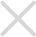# 合金风暴4.0

## 游戏截图## 简介

```《合金风暴》在这片魔法大陆上，邪恶势力始终威胁着世界的安宁，你作为屠魔者，承担起了这份责任，但与恶魔一起出现的合金宝藏也诱惑着世人，因此纷争的风暴已然刮起。

```

```【VIP】
VIP等级金额（元）累计
0=0
1=5
2=35
3=96
4=228
5=488
6=988
7=1988
8=3600
9=5800
10=9800
11=15000
12=21000
13=28000
14=36000
15=45000
16=55000
17=72000
18=98000
19=128000
20=198000```

# 相关游戏推荐

1每天上新近百款新游，尝鲜不容错过；

2第一时间更新海内外游戏大作；

3不同机型兼容测试，告别闪退；

4许愿想玩的游戏，小编立马上线；

{{title}}
{{ submit }}
{{ submit }}